### Home > CALC > Chapter 5 > Lesson 5.2.2 > Problem5-71

5-71.
1. Write an expression for the distance between each set of points. Homework Help ✎

1. (5, 10) and (8, 14)

2. (a, b) and (3, 5)

3. (85, −10) and (−8, 142)

4. A random point on y = x2 and (3, 5), (in terms of x.)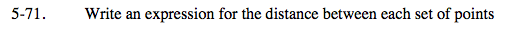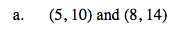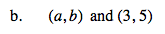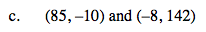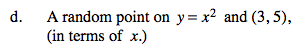The coordinates are (3, 5) and (x, x²).